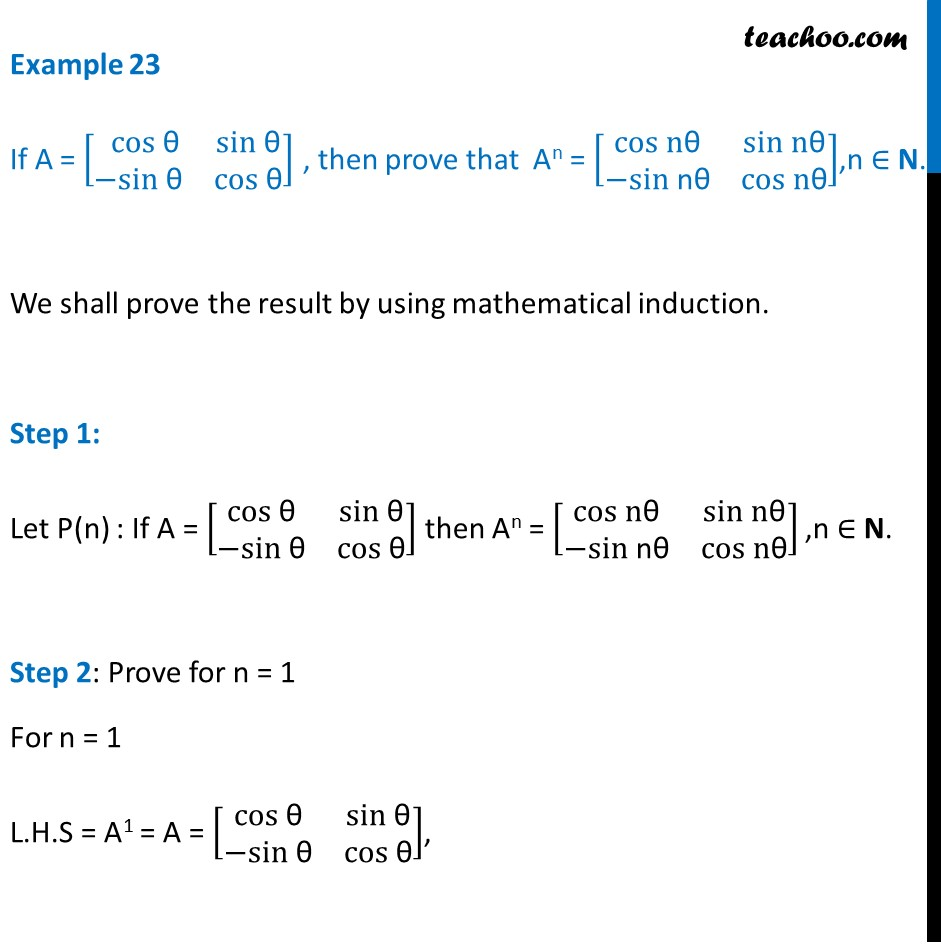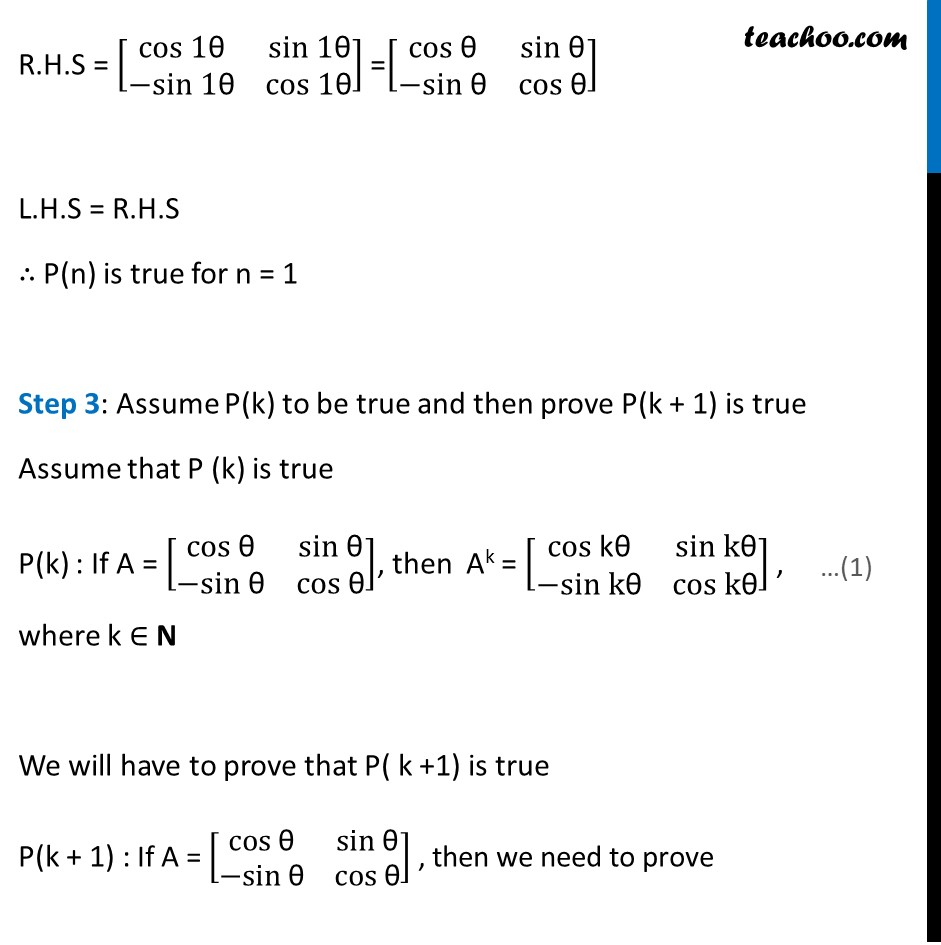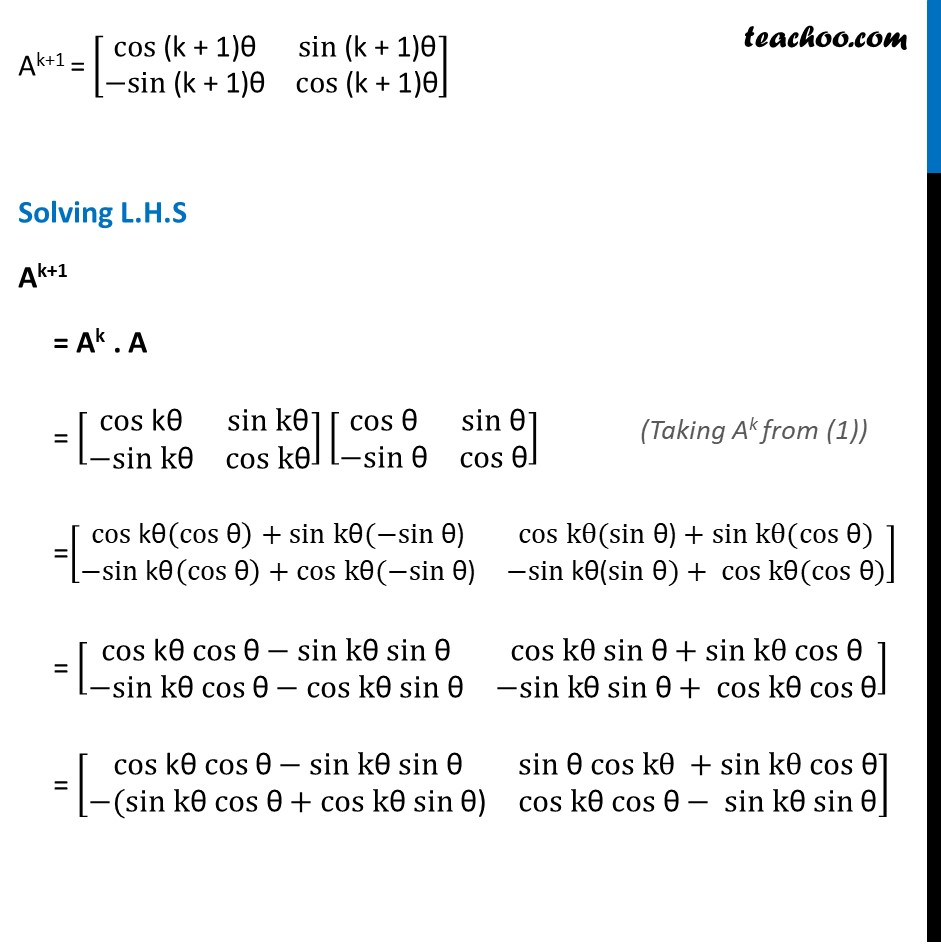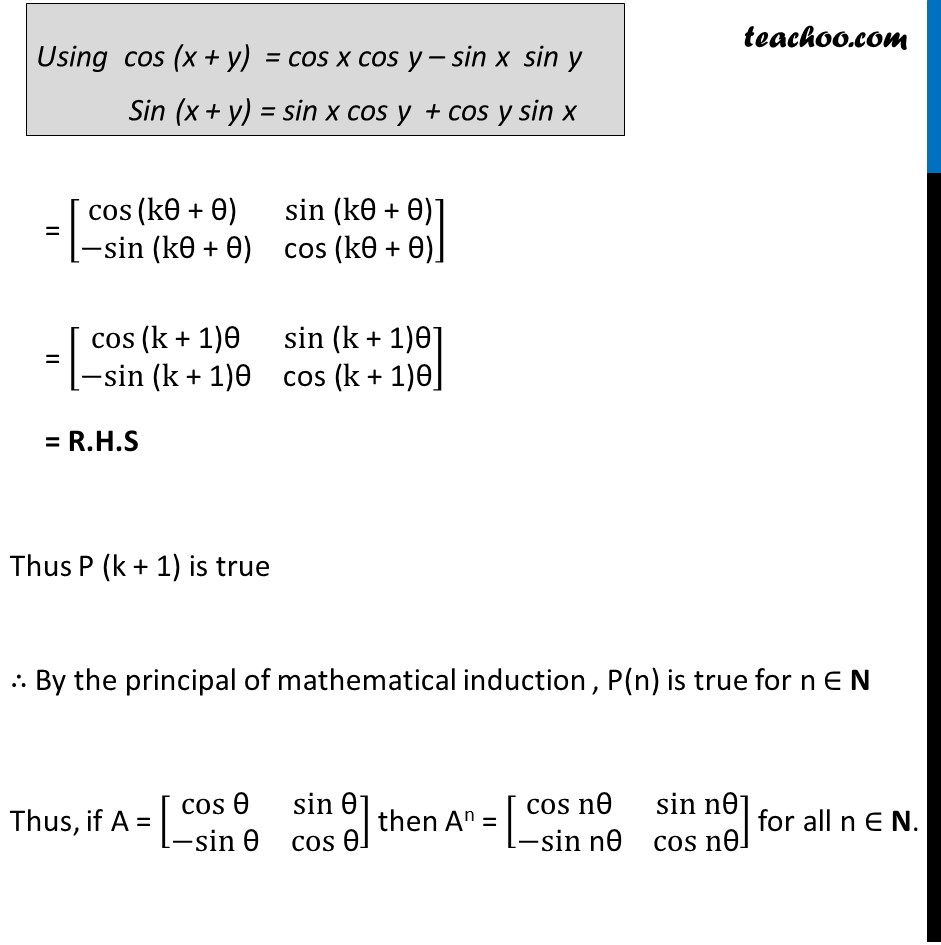Examples

Chapter 3 Class 12 Matrices
Serial order wiseLearn in your speed, with individual attention - Teachoo Maths 1-on-1 Class

### Transcript

Example 23 If A = [■8( cos" θ" &sin" θ" @−sin "θ" &cos" θ" )] , then prove that An = [■8(cos n"θ" &sin n"θ" @−sin "nθ" &cos n"θ" )],n ∈ N. We shall prove the result by using mathematical induction. Step 1: Let P(n) : If A = [■8(cos "θ" &sin" θ" @−sin "θ" &cos" θ" )] then An = [■8(cos n"θ" &sin n"θ" @−sin" nθ" &cos n"θ" )] ,n ∈ N. Step 2: Prove for n = 1 For n = 1 L.H.S = A1 = A = [■8(cos" θ" &sin" θ" @−sin" θ" &cos" θ" )], R.H.S = [■8(cos" 1θ" &sin "1θ" @−sin 1"θ" &cos "1θ" )] =[■8(cos" θ" &sin" θ" @−sin" θ" &cos" θ" )] L.H.S = R.H.S ∴ P(n) is true for n = 1 Step 3: Assume P(k) to be true and then prove P(k + 1) is true Assume that P (k) is true P(k) : If A = [■8(cos "θ" &sin "θ" @−sin "θ" &cos "θ" )], then Ak = [■8(cos "kθ" &sin k"θ" @−sin k"θ" &cos k"θ" )] , where k ∈ N We will have to prove that P( k +1) is true P(k + 1) : If A = [■8(cos" θ" &sin" θ" @−sin" θ" &cos" θ" )] , then we need to prove Ak+1 = [■8(cos" (k + 1)θ" &sin" (k + 1)θ" @−sin" (k + 1)θ" &cos" (k + 1)θ" )] Solving L.H.S Ak+1 = Ak . A = [■8(cos "kθ" &sin k"θ" @−sin k"θ" &cos k"θ" )] [■8(cos "θ" &sin "θ" @−sin "θ" &cos" θ" )] =[■8(cos" kθ" (cos" θ" )+sin k"θ" (−sin" θ)" &cos kθ(sin "θ)" +sin kθ(cos" θ" )@−sin" kθ" (cos "θ" )+cos k"θ" (−sin "θ)" &−sin "kθ(" sin "θ" )+ cos k"θ" (cos "θ" ))] = [■8(cos" kθ" cos" θ" −sin k"θ" sin" θ" &cos kθ sin "θ" +sin kθ cos" θ" @−sin" kθ" cos "θ" −cos k"θ" sin "θ" &−sin "kθ" sin "θ" + cos k"θ" cos "θ" )] = [■8(cos" kθ" cos" θ" −sin k"θ" sin" θ" &sin "θ" cos kθ +sin kθ cos" θ" @−(sin" kθ" cos "θ" +cos k"θ" sin "θ)" &cos k"θ" cos "θ" − sin "kθ" sin "θ" )] = [■8(cos⁡"(" k"θ + θ)" &sin "(" k"θ + θ)" @−sin" (" k"θ + θ)" &"cos (" k"θ + θ)" )] = [■8(cos⁡"(" k" + 1)θ" &sin" (" k" + 1)θ" @−sin "(" k" + 1)θ" &"cos (" k" + 1)θ" )] = R.H.S Thus P (k + 1) is true ∴ By the principal of mathematical induction , P(n) is true for n ∈ N Thus, if A = [■8(cos "θ" &sin" θ" @−sin "θ" &cos" θ" )] then An = [■8(cos n"θ" &sin n"θ" @−sin" nθ" &cos n"θ" )] for all n ∈ N.